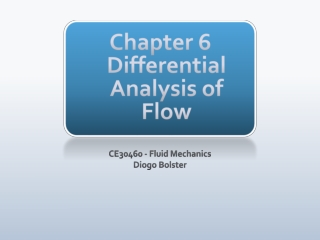DownloadDownload PresentationChapter 6 Differential Analysis of Flow

# Chapter 6 Differential Analysis of Flow

Télécharger la présentation## Chapter 6 Differential Analysis of Flow

- - - - - - - - - - - - - - - - - - - - - - - - - - - E N D - - - - - - - - - - - - - - - - - - - - - - - - - - -
##### Presentation Transcript

1. Chapter 6Differential Analysis of Flow CE30460 - Fluid Mechanics Diogo Bolster

2. Chapter Goals • Kinematics of given flow field • Continuous Continuity Equation • Navier-Stokes and Specific Solutions • Concepts of Potential Flow

3. Kinematics • Recall Material Derivative (LagrangianvsEulerian) • Acceleration of a fluid element

4. Types of Motion • Translation • Linear Deformation • Rotation • Angular Deformation • Draw each of these for a rectangular initial element….

5. Types of Motion

6. Linear Motion and Deformation

7. In 3 dimensions • Volumetric Dilatation Rate

8. Angular Motion and Deformation

9. Vorticity (Rotation) • Counterclockwise rotation is positive (z component is component out of the page). Others, in x-y also exist • vorticity (zero => irrotational) • Related, rate of shearing strain

10. Sample Problem 1

11. Sample Problem 2

12. Conservation of Mass But now in the limit of zero volume (to obtain differential form)

13. Forms of Continuity Equation • General Form • Steady • Incompressible

14. Cylindrical Polar Coordinates • General Form • Steady • Incompressible

15. Streamfunction • For incompressible, plane two dimensional flow we can define a streamfunction psi, such that • Quantifies the flow rate between two streamlines (lines of constant psi)

16. In cylindrical polar coordinates

17. Sample Problem 1

18. Sample Problem 2